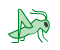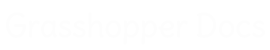﻿ Libra - Addon for Grasshopper | Grasshopper DocsCommunity documentation for Grasshopper add-ons & plugins# Libra

##### Libra is a Grasshopper plug-in which implements a topology grammar for generating structural topologies. Starting from an incomplete network, namely (a) the geometric boundaries that a network grows into and (b) the applied external forces, Libra operates an incremental transformative process that allows the transition to a complete network. In other words, the incremental elimination of interim forces in the network. At every intermediate step the network is in static equilibrium (interim when interim forces are still present, or global when no interim forces exist).
 Domain
 Forces
 Model
 Transform
 Xploration

### Model

 Construct Model (ConstructModel)Construct an interim model with domain (geometric boundaries) Model Export to Illustrator (ModelExport)Model export to Adobe Illustrator Model Geometry (ModelGeometry)Extract the model geometry Model History (ModelHistory)Extract transformation history Model Metrics (text) (ModelMetrics)The model metrics as text Model Metrics (spider graph) (ModelSpiderGraph)The model metrics represented as spider graph Model Undo (UndoModel)Undo transformations on model Select Force (SelectForce)Retrieves a force from the Form Diagram

### Transform

 Apply Transformation Policy (Transform)Apply transformation policy onto an interim model Construct Node Placement Rule (NodePRule)Construct a node placement rule Construct Transformation Policy (Policy)Construct a transformation policy Construct Force Indeterminacies Rule (ForceIRule)Construct a force indeterminacies rule Construct Force Selection Rule (ForceSRule)Construct a force selection rule Construct Transformation Policy (fast) (PolicyFast)Construct a fast transformation policy

### Forces

 Construct Force Set (ConstructForceSet)Select forces and construct a Monomial/Binomial/Trinomial Deconstruct Force Set (DeconstructForceSet)Deconstruct force set Force Set Domains (ForceSetDomains)Get the domains of a force set Visualize Force Set (VisualizeForceSet)Visualize a force set by highlighting its forces

### Domain

 Construct Domain (ConstructDomain)Construct a domain of any type Deconstruct Domain (DeconstructDomain)Deconstruct a domain of any type Visualize Domain (VisualizeDomain)Visualize a domain

### Xploration

 Design Space Exploration (DSE+IGA)Equilibrium-based Design Space Exploration (DSE) through policies, rules and interactive genetic algorithms(IGA) Design Space Exploration Reader (DSEReader)Design Space Exploration (DSE) Reader component to retrieve every single design candidate that was generated

Site design © Robin Rodricks.   Libra and associated data © 2023 Ioannis Mirtsopoulos.
Rhinoceros and Grasshopper are registered trademarks of Robert McNeel & Associates.  Hosted by GitHub